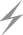Computer Aids for VLSI Design
Steven M. Rubin
Copyright © 1994

# Appendix A: Gerber Format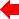Appendix 1 of 7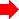This appendix describes the format of The Gerber Scientific Instrument Company's photoplotters. Although Gerber is only one of the manufacturers of photoplotters, many other companies have used Gerber format for their photoplotters, making it somewhat of an industry standard. Within the Gerber product line, there are many different models and they all read slight variations of this format. Some machines read a binary version of the format, some a text version, and some can accept either. For the sake of simplicity, only the common attributes of the text format will be described. More detail can be obtained from the Gerber Scientific Instrument Company, 83 Gerber Road West, South Windsor, Connecticut 06074.

## A.1 Overall Format

Figure A.1 gives the BNF syntax of Gerber format. Each line of text contains a series of commands and their numeric parameters, terminated with an asterisk. A command consists of a single letter and the parameter is a number that immediately follows the letter. All numbers are specified with implied decimal points, such that a sequence of m + n digits forms a number that has m digits to the left of the decimal point and n digits to the right. It is possible to shorten a number to fewer than m + n digits by specifying that leading or trailing zeros are omitted. If, for example, m = 3, n = 2, and leading zeros are omitted, then the digits `12345` form the number 123.45 and the digits `400` represent the number 4.

 file ::= graphics | graphics file graphics ::= line | arc | parabola | cubic | text | misc line ::= firstline [ nextlines ] firstline ::= [ seq ] linetype coordinate nextlines ::= nextline | nextline nextlines nextline ::= [ seq ] coordinate linetype ::= `G12` | `G11` | `G01` | `G10` | `G60` arc ::= [ seq ] circletype `X` snum `Y` snum `I` num `J` num [ draft ] `*` circletype ::= `G02` | `G03` parabola ::= firstparabola [ nextparabolas ] firstparabola ::= [ seq ] `G06` coordinatepair nextparabolas ::= nextparabola | nextparabola nextparabolas nextparabola ::= [ seq ] coordinatepair coordinatepair ::= coordinate [ seq ] coordinate cubic ::= [ seq ] `G07` coordinatelist coordinatelist ::= coordinate | coordinate [ seq ] coordinatelist text ::= [ seq ] `G56` [ rotation ] [ scale ] font message `*` rotation ::= `W` [ + | - ] digit digit digit digit digit digit scale ::= `M50` | `M51` | `M52` | `M53` | `M54` font ::= `D10` message ::= messageletter | messageletter message messageletter ::= any letter but * misc ::= [ seq ] `G54` [ miscellaneous ] [ draft ] | [ seq ] drawtype `*` miscellaneous ::= `M00` | `M30` drawtype ::= `G90` | `G91` coordinate ::= `X` snum `Y` snum [ draft ] `*` draft ::= `D01` | `D02` | `D04` | `D05` | `D06` | `D07` seq ::= n digits snum ::= [ + | - ] digits num ::= digits digits ::= digit | digit digits digit ::= `0` | `1` | `2` | `3` | `4` | `5` | `6` | `7` | `8` | `9`
FIGURE A.1 Gerber format.

The most important command letter is the `G` command, which is called the preparatory function code. The number that follows the `G` sets a mode for the data that follow (see Fig. A.2). For example, `G57` is the comment command that allows documenting text to follow, up to the asterisk. There are six different drawing functions that these codes can introduce: lines, circular arcs, parabolas, cubic curves, text, and miscellaneous. In addition, the style of plotting coordinates can be declared with these preparatory function codes (absolute or relative).

 `G12` Line drawing, 0.01x scale `G11` Line drawing, 0.1x scale `G01` Line drawing, 1x scale `G10` Line drawing, 10x scale `G60` Line drawing, 100x scale `G02` Circular-arc drawing, clockwise `G03` Circular-arc drawing, counterclockwise `G06` Parabola drawing `G07` Cubic-curve drawing `G56` Text drawing `G54` Miscellaneous drawing `G57` Comment `G90` Absolute coordinates `G91` Relative coordinates
FIGURE A.2 Gerber format preparatory function codes.

Before the drawing operations are described, some background must be provided. The photoplotter writes in a random-access style by moving a pen to arbitrary locations on the film. This pen is actually a photographic writing head but the analogy to real pens is correct. When the pen is moved, it can be either up or down. Moving the pen while it is up simply causes the pen to be repositioned on the film. Moving the pen while it is down causes the pen to write. All the drawing commands presume that the pen is down.

## A.2 Lines

To draw lines, the preparatory function codes `G01`, `G10`, `G11`, `G12`, or `G60` must appear followed by coordinate information. For example, to draw a 3 × 3 square starting at the lower-left corner, the following codes can be used (presuming that m.n is 2.1):

```    G91*                G57 Set Relative Coordinates *
G01X030Y000*        G57 Draw bottom *
X000Y030*        G57 Draw right *
X-030Y000*       G57 Draw top *
X000Y-030*       G57 Draw left *
```
Notice that the preparatory function code does not have to be repeated to remain in effect. The `X` and `Y` commands specify the actual drawing coordinates. They can accept signed values but the number of digits must fit the m.n format.

The line-drawing commands can be used to position the pen without drawing. This is done by placing the pen-up drafting code `D02` on the drawing command line. Figure A.3 lists the possible drafting codes.

 `D01` Drawing on `D02` Drawing off `D04` Dashed lines Type 1 `D05` Dashed lines Off `D06` Dashed lines Type 2 `D07` Dashed lines Type 3 `D10` Standard font `D11` - `D99` Alternate fonts
FIGURE A.3 Gerber format drafting codes.

For example, to move the pen to location (12,-3) use the following command (assuming absolute coordinate mode and that m.n is 2.2):

`    G01X1200Y-0300D02*`

## A.3 Circular Arcs

Drawing circular arcs requires that a center, a starting point, and an ending point be specified. The current pen position is used as the arc starting point; the `X` and `Y` parameters specify the arc endpoint; and the `I` and `J` parameters specify the arc center. The direction of drawing the arc is taken from the particular arc command used: `G02` or `G03`. One restriction on the drawing of arcs is that they must start and end in the same quadrant. This is because the `I` and `J` parameters, which specify the arc center, are unsigned absolute distances relative to the starting point. Therefore the arc must remain in the same quadrant to prevent ambiguity in the center location. Drawing a complete circle requires that four of these commands be issued.FIGURE A.4 Circular arc must be drawn in two pieces when it crosses a quadrant boundary.

As an example of circular-arc drawing, suppose that the arc in Fig. A.4 is to be drawn. This arc crosses a quadrant boundary and so must be drawn in two pieces. Further assume that the m.n format is 2.2 and that the coordinate mode is absolute. These commands will draw the arc:

```    G01X-0400Y0300D02*           G57 Move to (-4, 3) *
G02X0000Y0500I0400J0300D01*  G57 Draw to (0, 5) *
X0300Y0400J0500*          G57 Draw to (3, 4) *
```
Notice that the second line does not have an `I` command because the value is zero, which is the default.

The restriction of drawing only one quadrant at a time could be lifted if the `I` and `J` commands had signs. Then the circle center could be unambiguously specified and the quadrant would not be needed as a clue to the photoplotter. Some photoplotters do allow signs in the arc-center specification and these plotters can draw full circles at once. However, for the sake of compatibility, use of this option is not advised. A CAD system can break a circle into quadrants with little effort and the resulting description will not be significantly larger.

## A.4 Curves

Parabolic curves can be specified by providing a start point, an endpoint, and a vertex through which the curve must pass. The start point is the current point; the vertex is specified by the first set of `X` and `Y` coordinates on that line; and the endpoint is specified by a second set of `X` and `Y` coordinates on the next line.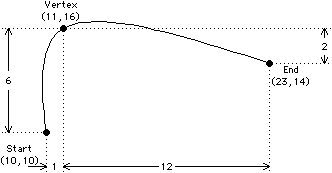FIGURE A.5 Parabolic arc.

For example, to draw the parabola in Fig. A.5, assuming relative coordinates, a current pen position of (10,10), and m.n is 2.0, use:

```    G06X01Y06*    G57 The vertex *
X12Y-02*   G57 The endpoint *
```
Note that it takes two lines in the file to draw the parabola and that the second line has no `G` code. The preparatory function code for parabolas sets a mode so that every pair of lines after the first parabola will be interpreted as another parabola until a different preparatory function code is introduced.

Cubic, or third-order, curves can be generated by giving a sequence of four or more points. Parabolic curve fitting is used on the ends and cubic fitting is used in the middle segments. For example, to draw the five-point curve in Fig. A.6, the following commands can be used (assuming absolute coordinates, m.n is 3.0 and current pen position is (3,5)):

```    G07X008Y009f*    G57 Draw segment A *
X015Y004*     G57 Draw segment B *
X022Y007*     G57 Draw segment C *
X026Y006*     G57 Draw segment D *
```
The two end segments (A and D) will be drawn using the three endpoints in a parabolic curve, but the middle two segments (B and C) will be drawn using the four surrounding points in a cubic curve.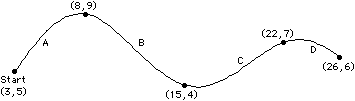FIGURE A.6 Cubic curve.

## A.5 Text

Text can be drawn using the `G56` preparatory function code. Following this code are two optional modifier codes, a nonoptional font code, and the text string to draw. All text is drawn so that the lower-left corner of the string is at the current pen position.

The first optional modifier of text is the `W` code, which specifies a rotation for the string. Following the `W` is an optional sign and a number in 3.3 format (that is, six digits with the implied decimal in the middle). This is a rotation in degrees counterclockwise from normal, rotated about the current pen position. Thus text rotated 90 degrees will read from bottom to top rather than from left to right.

The second optional modifier is the scaling factor, which is specified as an `M` code (see Fig. A.7) followed by a scaling number. The unscaled size of text depends on the plotting unit and is 0.015 inches square if plotting in inches or 1.5 millimeters square if plotting in millimeters.

 `M00` Program stop `M30` Rewind tape `M50` Scale text by 10 `M51` Scale text by 25 `M52` Scale text by 50 `M53` Scale text by 75 `M54` Scale text by 100
FIGURE A.7 Miscellaneous Gerber commands.

The text font code specifies a character set to use when drawing text. This code is not optional and should usually be the drafting code `D10` because font 10 is the only standard font. The text to be plotted follows the `D10` immediately and proceeds up to the asterisk at the end of the line.

Some examples of text usage are in order. Figure A.8 shows the results of the following command (assuming that the current pen position is (5,7) and that the drawing units are inches):
```    G56M52D10HELLO THERE*
```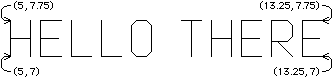FIGURE A.8 Text plotting.
Figure A.9 shows the result of the following command (assuming that the current pen position is (6,8) and that the drawing units are millimeters):
```    G56W-090000D10AMY*
```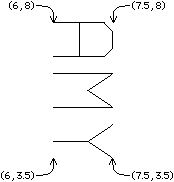FIGURE A.9 Text plotting.

## A.6 Miscellaneous

The final preparatory function code is `G54`, which performs miscellaneous control. Following that code is a `D` (drafting) code from Fig. A.3. These codes are used to raise or lower the pen and to select a pen drawing style. Notice that this code also selects the text font. Most usage of the drafting code is incorporated into other preparatory function codes. However, if the drafting code is to be issued independently of drawing, that can be done with the `G54` code.
For example, to draw the symbol in Fig. A.10, use these commands (assuming that the current position is (5,5), the drawing mode is relative, and the m.n is 2.0):
```    G01X05Y05*        G57 Lower-left to upper-right *
X00Y-05D02*    G57 Move down without drawing *
X-05Y05D04*    G57 Lower-right to upper-left (dashed) *
G54D05*           G57 Turn off dashed lines *
```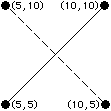FIGURE A.10 Drawing example.

In addition to the preparatory function codes, a sequencing code can be used to order the lines in a file. The `N` command is used for sequencing followed by any digits (up to three) that form a sequence number. This number is not used by the plotter and is for organizational purposes only. Presumably, it dates back to the days of punched cards, when a dropped deck had to be reordered. Most data transfer is done electronically these days so the sequence data is unnecessary.

There are many other options in Gerber format. However, most of them are restricted to certain plotter models and others pertain to obscure tasks. In general, task-specific parameters are set up before a plot is done. These parameters describe overall scaling, character fonts, dashed line styles, digit format (m.n), absolute or relative coordinates, and metric or English units. Some parameters can be changed during the plot but most are constant and apply uniformly to the task at hand.Previous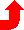Table of Contents NextSteven M. Rubin Static Free Software